#### Graph representations of a plan

The game against nature involves two decision makers: nature and the robot. Once the plan is formulated, the decisions of the robot become fixed, which leaves nature as the only remaining decision maker. Using this interpretation, a directed graph can be defined in the same way as in Section 2.1, except nature actions are used instead of the robot's actions. It can even be imagined that nature itself faces a discrete feasible planning problem as in Formulation 2.1, in which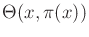replaces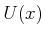, and there is no goal set. Let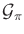denote a plan-based state transition graph, which arises under the constraint that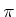is executed. The vertex set ofis. A directed edge inexists fromto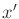if there exists some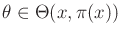such that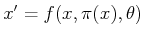. Thus, from each vertex in, the set of outgoing edges represents all possible transitions to next states that are possible, given that the action is applied according to. In the case of probabilistic uncertainty,becomes a weighted graph in which each edge is assigned the probability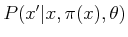. In this case,corresponds to the graph representation commonly used to depict a Markov chain.

A nondeterministic forward projection can easily be derived fromby following the edges outward from the current state. The outward edges lead to the states of the one-stage forward projection. The outward edges of these states lead to the two-stage forward projection, and so on. The probabilistic forward projection can also be derived from.

Steven M LaValle 2020-08-14